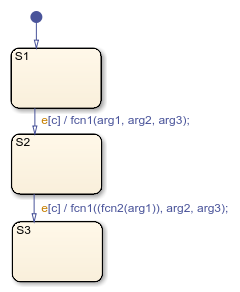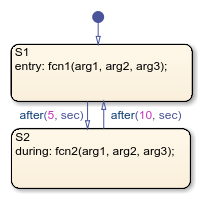Main Content

## Call C Library Functions in C Charts

Stateflow® charts in Simulink® models have an action language property that defines the syntax for state and transition actions. An icon in the lower-left corner of the chart canvas indicates the action language for the chart.

•MATLAB® as the action language.

•C as the action language.

### Call C Library Functions

You can call this subset of the C Math Library functions:

 ```abs* **``` `acos**` `asin**` `atan**` `atan2**` `ceil**` `cos**` `cosh**` `exp**` `fabs` `floor**` `fmod**` `labs` `ldexp**` `log**` `log10**` `pow**` `rand` `sin**` `sinh**` `sqrt**` `tan**` `tanh**` * The Stateflow `abs` function goes beyond that of its standard C counterpart with its own built-in functionality. For more information, see Call the abs Function. ** You can also replace calls to the C Math Library with application-specific implementations for this subset of functions. For more information, see Replacement of Math Library Functions with Application Implementations.

When you call these functions, double precision applies unless all the input arguments are explicitly single precision. When a type mismatch occurs, a cast of the input arguments to the expected type replace the original arguments. For example, if you call the `sin` function with an integer argument, a cast of the input argument to a floating-point number of type `double` replaces the original argument.

Note

Because the input arguments to the C library functions are first cast to floating-point numbers, function calls with arguments of type `int64` or `uint64` can result in loss of precision.

If you call other C library functions not listed above, include the appropriate `#include...` statement in the Simulation Target pane of the Configuration Parameters.

### Call the abs Function

Interpretation of the Stateflow `abs` function goes beyond the standard C version to include integer and floating-point arguments of all types as follows:

• If `x` is an integer of type `int32` or `int64`, the standard C function `abs` applies to `x`, or `abs(x)`.

• If `x` is an integer of type `int16` or `int8`, the standard C `abs` function applies to a cast of `x` as an integer of type `int32`, or `abs((int32)x)`.

• If `x` is a floating-point number of type `double`, the standard C function `fabs` applies to `x`, or `fabs(x)`.

• If `x` is a floating-point number of type `single`, the standard C function `fabs` applies to `a` cast of `x` as a `double`, or `fabs((double)x)`.

• If `x` is a fixed-point number, the standard C function `fabs` applies to a cast of the fixed-point number as a `double`, or `fabs((double) Vx)`, where V`x` is the real-world value of `x`.

If you want to use the `abs` function in the strict sense of standard C, cast its argument or return values to integer types. See Type Cast Operations.

Note

If you declare `x` in custom code, the standard C `abs` function applies in all cases. For instructions on inserting custom code into charts, see Reuse Custom Code in Stateflow Charts.

### Call min and max Functions

You can call `min` and `max` by emitting the following macros automatically at the top of generated code.

```#define min(x1,x2) ((x1) > (x2) ? (x2):(x1)) #define max(x1,x2) ((x1) > (x2) ? (x1):(x2))```

To allow compatibility with user graphical functions named `min()` or `max()`, generated code uses a mangled name of the following form: `<prefix>_min`. However, if you export `min()` or `max()` graphical functions to other charts in your model, the name of these functions can no longer be emitted with mangled names in generated code and conflict occurs. To avoid this conflict, rename the `min()` and `max()` graphical functions.

### Replacement of Math Library Functions with Application Implementations

You can configure the code generator to change the code that it generates for math library functions such that the code meets application requirements. To do this you configure the code generator to apply a code replacement library (CRL) during code generation. If you have an Embedded Coder® license, you can develop and apply custom code replacement libraries.

For more information about replacing code, using code replacement libraries that MathWorks® provides, see What Is Code Replacement? (Simulink Coder) and Code Replacement Libraries (Simulink Coder). For information about developing custom code replacement libraries, see What Is Code Replacement Customization? (Embedded Coder) and Code You Can Replace From Simulink Models (Embedded Coder).

### Call Custom C Code Functions

You can specify custom code functions for use in C charts for simulation and C code generation.

#### Specify Custom C Functions for Simulation

To specify custom C functions for simulation:

1. Open the Model Configuration Parameters dialog box.

2. Select Simulation Target.

3. Specify your custom C files, as described in Access Custom C Code in Nonlibrary Charts.

#### Specify Custom C Functions for Code Generation

To specify custom C functions for code generation:

1. Open the Model Configuration Parameters dialog box.

2. Select Code Generation > Custom Code.

3. Specify your custom C files, as described in Integrate External Code for All Charts (Simulink Coder).

#### Guidelines for Calling Custom C Functions in Your Chart

• Define a function by its name, any arguments in parentheses, and an optional semicolon.

• Pass parameters to user-written functions using single quotation marks. For example, `func('string')`.

• An action can nest function calls.

• An action can invoke functions that return a scalar value (of type `double` in the case of MATLAB functions and of any type in the case of C user-written functions).

#### Guidelines for Writing Custom C Functions That Access Input Vectors

• Use the `sizeof` function to determine the length of an input vector.

For example, your custom function can include a for-loop that uses `sizeof` as follows:

```for(i=0; i < sizeof(input); i++) { ...... }```
• If your custom function uses the value of the input vector length multiple times, include an input to your function that specifies the input vector length.

For example, you can use `input_length` as the second input to a `sum` function as follows:

```int sum(double *input, double input_length) ```

Your `sum` function can include a for-loop that iterates over all elements of the input vector:

```for(i=0; i < input_length; i++) { ...... }```

#### Function Call in Transition Action

Example formats of function calls using transition action notation appear in the following chart.A function call to `fcn1` occurs with `arg1`, `arg2`, and `arg3` if the following are true:

• `S1` is active.

• Event `e` occurs.

• Condition `c` is true.

• The transition destination `S2` is valid.

The transition action in the transition from `S2` to `S3` shows a function call nested within another function call.

#### Function Call in State Action

Example formats of function calls using state action notation appear in the following chart.Chart execution occurs as follows:

1. When the default transition into `S1` occurs, `S1` becomes active.

2. The `entry` action, a function call to `fcn1` with the specified arguments, executes.

3. After 5 seconds of simulation time, `S1` becomes inactive and `S2` becomes active.

4. The `during` action, a function call to `fcn2` with the specified arguments, executes.

5. After 10 seconds of simulation time, `S2` becomes inactive and `S1` becomes active again.

6. Steps 2 through 5 repeat until the simulation ends.

#### Pass Arguments by Reference

A Stateflow action can pass arguments to a user-written function by reference rather than by value. In particular, an action can pass a pointer to a value rather than the value itself. For example, an action could contain the following call:

```f(&x); ```

where `f` is a custom-code C function that expects a pointer to `x` as an argument.

If `x` is the name of a data item defined in the Stateflow hierarchy, the following rules apply:

• Do not use pointers to pass data items input from a Simulink model.

If you need to pass an input item by reference, for example, an array, assign the item to a local data item and pass the local item by reference.

• If `x` is a Simulink output data item having a data type other than `double`, the chart property Use Strong Data Typing with Simulink I/O must be on (see Specify Properties for Stateflow Charts).

• If the data type of `x` is `boolean`, you must turn off the coder option Use bitsets for storing state configuration.

• If `x` is an array with its first index property set to 0 (see Set Data Properties), then you must call the function as follows.

`f(&(x));`

This passes a pointer to the first element of `x` to the function.

• If `x` is an array with its first index property set to a nonzero number (for example, 1), the function must be called in the following way:

`f(&(x))`;

This passes a pointer to the first element of `x` to the function.

## SupportGet trial now